# OECD Discriminatory family code data analysis 3 - average and confidence intervals using RPhoto by Alexander Schimmeck on Unsplash

This is following of above post.
In this post, I will calculating confidence intervals for "atwm"; Attitudes Towards Working Mothers and "em",; Early Marriage.

Let's begin.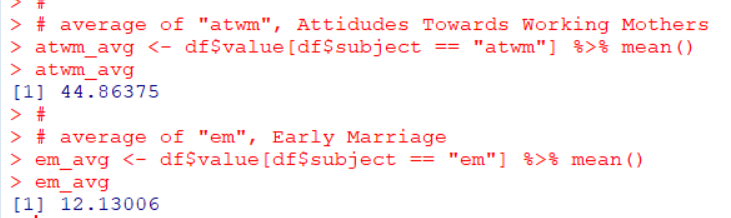We can use mean() function to calculate average. "atwm" average is 44.8 and "em" average is 12.1.

To calculate confidence intervals, we need average and followings

--- number of observations

--- standard deviation of observations

--- standard error of observations

--- confidence level, I use 5%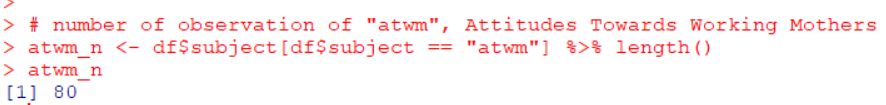I use lenght() function to get number of observations. "atwm" number of observations is 80.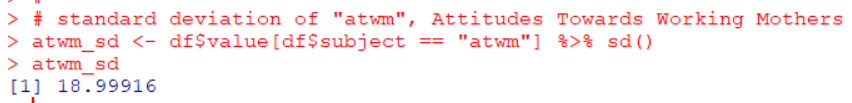sd() function gets standard deviation. "atwm" standard deviation is 18.999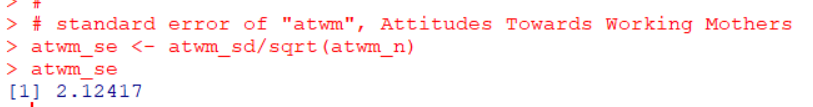Standard error is "standard deviation / sqrt(number of observations)". So the smaller standard deviation, the smaller standard error, the larger number of observations, the smaller standard error.

I use confidence level 5%, it means qt(0.975, n - 1).

Let's calculate confidence intervals for "atwm"; Attitudes Towards Working Mothers.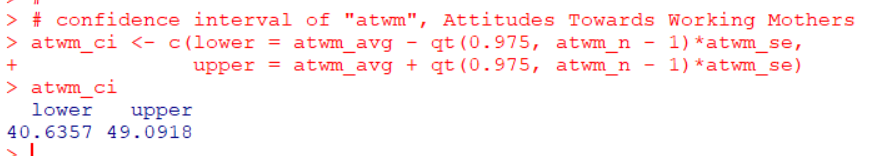Confidence Intervals is 40,64 ~ 49.09 at 5% level.

Next, I will calculate confidence intervals for "em"; Early Marriage.
Before calculate it, I will make custom function with function() for calculating confidence intervals.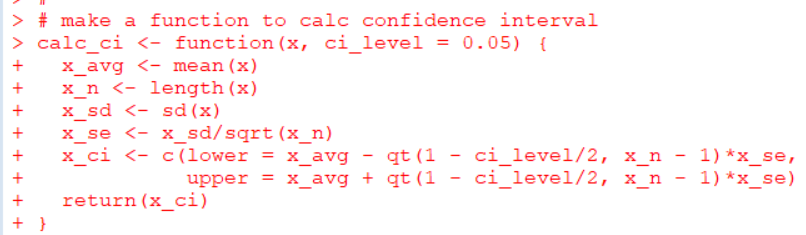Let's check if this function will do.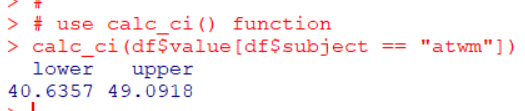This result is the same as previous result. So, this calc_ci() is working correctly.

I use calc_ci() to get confidence intervals for "em"; Early Marriage.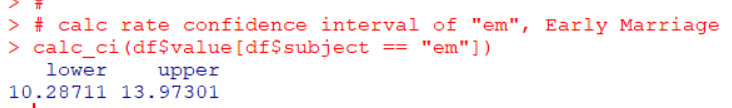"em"; Early Marriage confidence intervals at 5% level is 10.28 ~ 13.97.

Lastly, I make a plot to show average value and confidence intervals.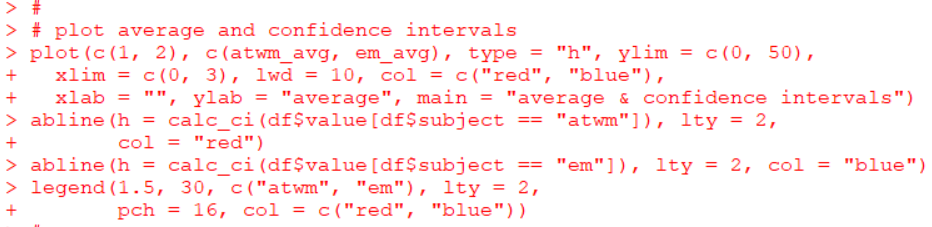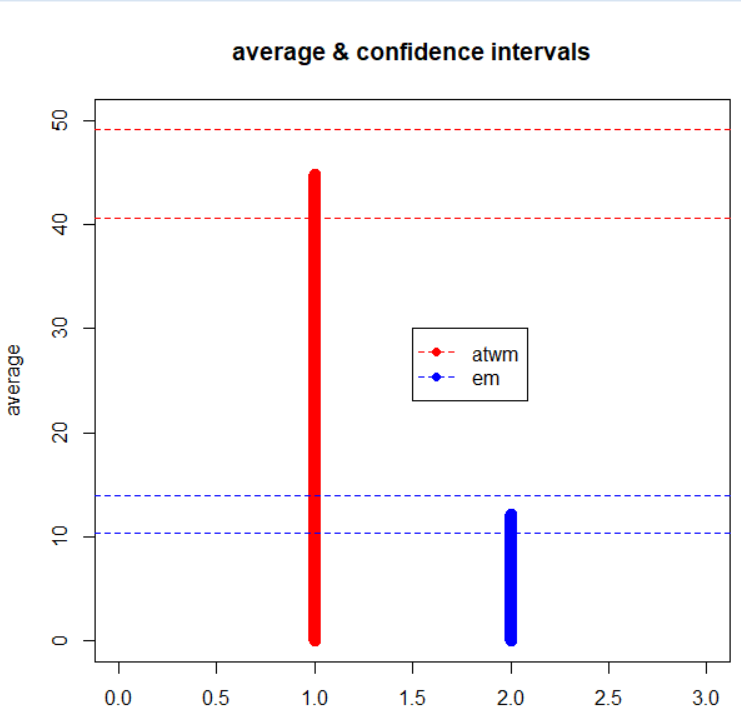I use plot() functuon with type = "h" and abline().
That's it. Thank you!

Next post is